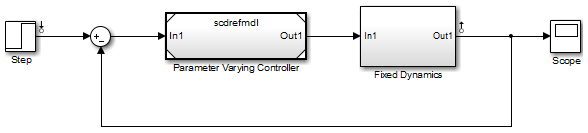linlftfold

Combine linearization results from specified blocks and model

Syntax

lin = linlftfold(lin_fixed,blocksubs)

Description

lin = linlftfold(lin_fixed,blocksubs) combines the following linearization results into one linear model lin:

• Linear model lin_fixed, which does not include the contribution of specified blocks in your Simulink® model.

Compute lin_fixed using linlft.

• Block linearizations for the blocks excluded from lin_fixed

You specify the block linearizations in a structure array blocksubs, which contains two fields:

• 'Name' is a character vector or string specifying the block path of the Simulink block to replace.

• 'Value' is the value of the linearization for each block.

Examples

collapse all

In this example, the scdtopmdl model contains two subsystems in the feedforward path.

• Fixed portion, which contains everything except the Parameter Varying Controller model reference

• Parameter Varying Controller model, which references the scdrefmdl model

Open the top-level model.

topmdl = 'scdtopmdl';
open_system(topmdl)Linearize this model without the Parameter Varying Controller block.

io = getlinio(topmdl);
blocks = {'scdtopmdl/Parameter Varying Controller'};
sys_fixed = linlft(topmdl,io,blocks);

Linearize the controller model.

refmdl = 'scdrefmdl';
sys_pv = linearize(refmdl);

Combine the linearization results.

BlockSubs(1) = struct('Name',blocks{1},'Value',sys_pv);